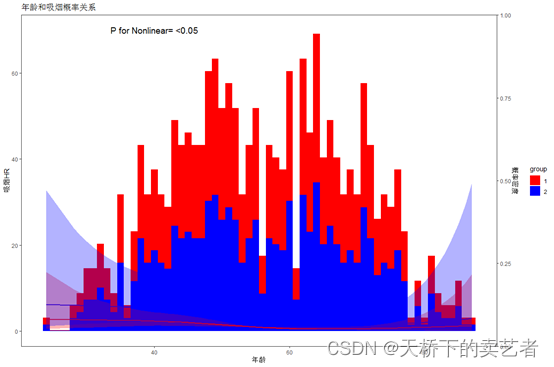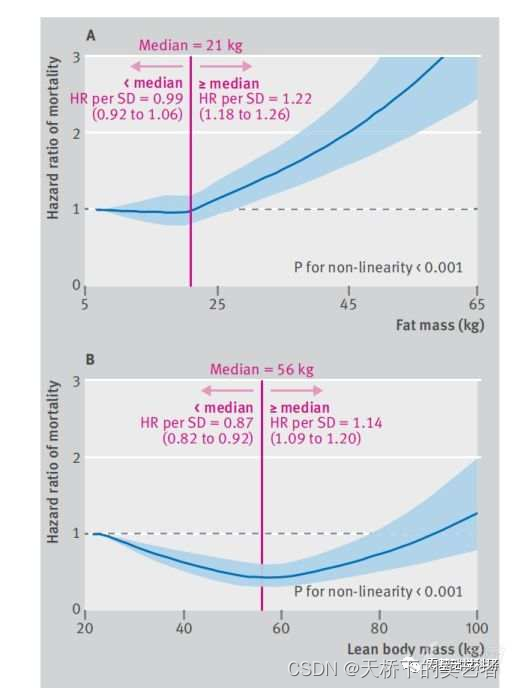# ggrcs包2.4版本上线: 一个用于绘制直方图+限制立方样条+双坐标轴图的R包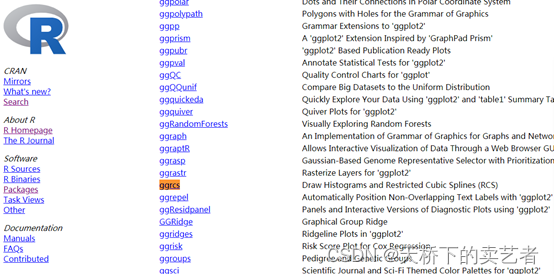``````install.packages("ggrcs")
``````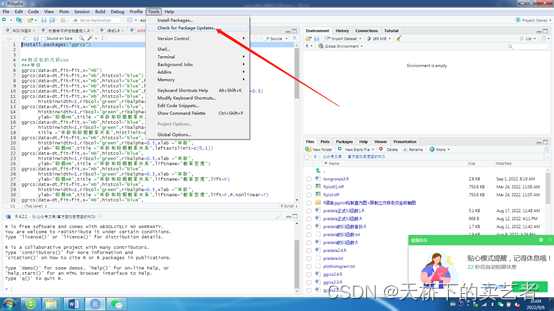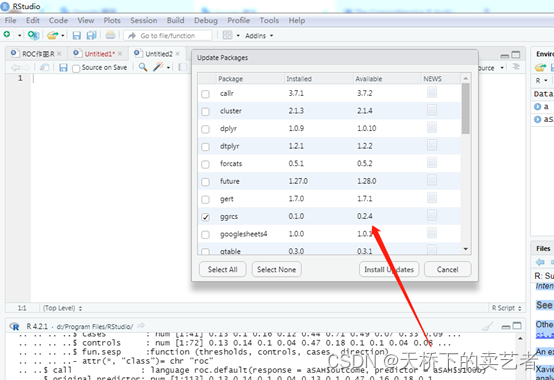``````library(rms)
library(ggplot2)
library(scales)
library(ggrcs)
dt<-smoke
fit<- cph(Surv(time,status==1) ~ rcs(age,4)+gender, x=TRUE, y=TRUE,data=dt)
``````

``````ggrcs(data=dt,fit=fit,x="age")
``````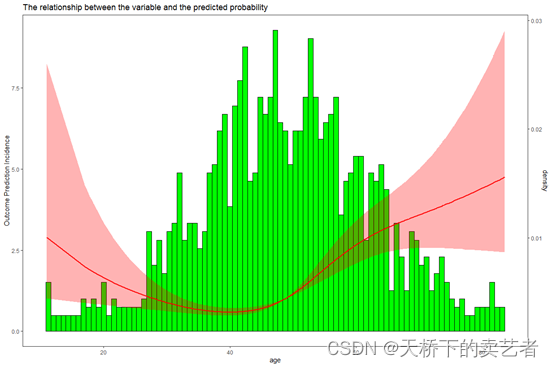``````ggrcs(data=dt,fit=fit,x="age",histcol="blue",
histbinwidth=1,ribcol="green",ribalpha=0.5,xlab ="年龄",
ylab="吸烟HR",title ="年龄和吸烟概率关系",liftname="概率密度",lift=F)
``````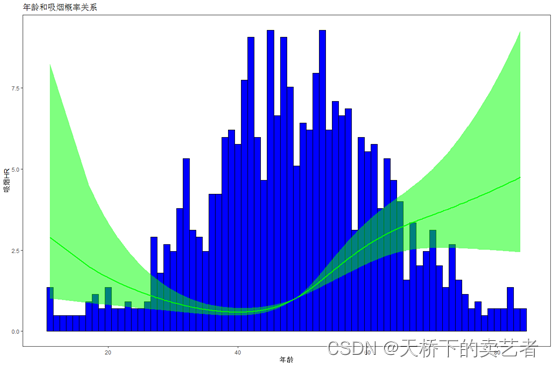``````ggrcs(data=dt,fit=fit,x="age",histcol="blue",
histbinwidth=1,ribcol="green",ribalpha=0.5,xlab ="年龄",
ylab="吸烟HR",title ="年龄和吸烟概率关系",liftname="概率密度",lift=F,P.Nonlinear=T)
``````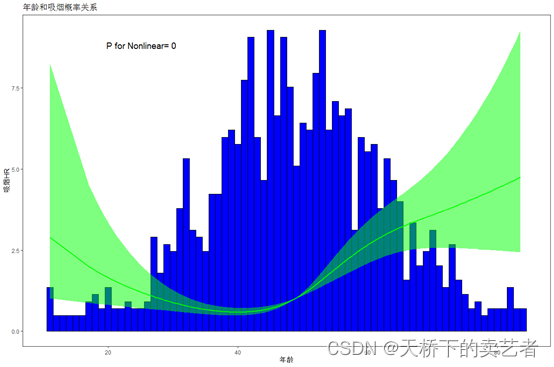``````ggrcs(data=dt,fit=fit,x="age",histcol="blue",
histbinwidth=1,ribcol="green",ribalpha=0.5,xlab ="年龄",
ylab="吸烟HR",title ="年龄和吸烟概率关系",
liftname="概率密度",lift=F,P.Nonlinear=T,Pvalue="<0.05")
``````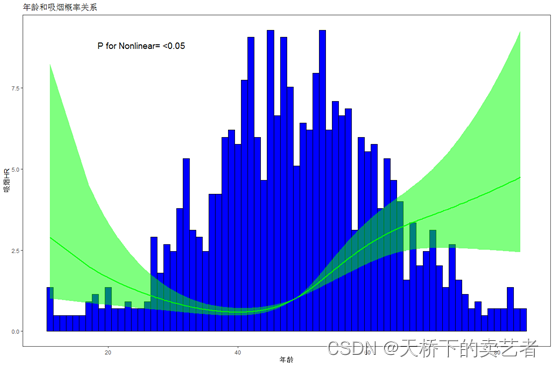``````p<-ggrcs(data=dt,fit=fit,x="age",histcol="blue",
histbinwidth=1,ribcol="green",ribalpha=0.5,xlab ="年龄",
ylab="吸烟HR",title ="年龄和吸烟概率关系",
liftname="概率密度",lift=F,P.Nonlinear=T,Pvalue="<0.05")
p+geom_vline(aes(xintercept=45.6),colour="red", linetype=1)
``````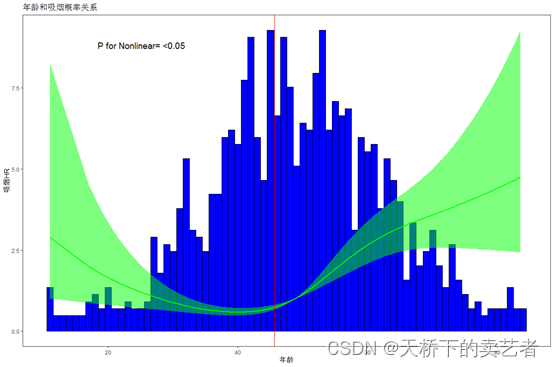``````ggrcs(data=dt,fit=fit,x="age",group="gender")
``````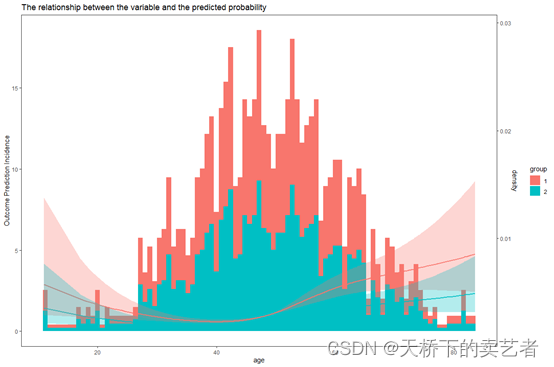``````ggrcs(data=dt,fit=fit,x="age",group="gender",groupcol=c("red","blue"),histbinwidth=1)
``````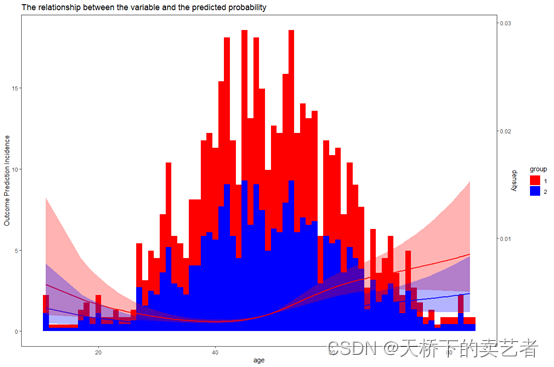``````ggrcs(data=dt,fit=fit,x="age",group="gender",
groupcol=c("red","blue"),histbinwidth=1,ribalpha=0.5,xlab ="年龄",
ylab="吸烟HR",title ="年龄和吸烟概率关系",leftaxislimit=c(0,1),liftname="概率密
``````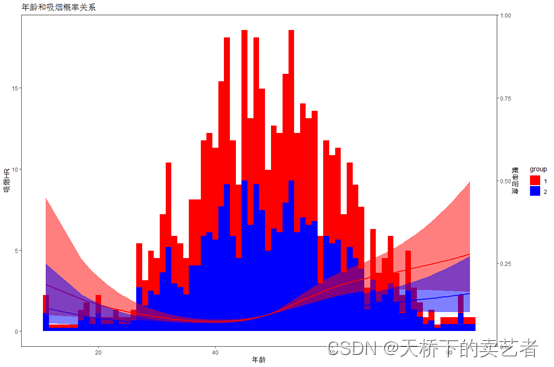``````ggrcs(data=dt,fit=fit,x="age",group="gender",
groupcol=c("red","blue"),histbinwidth=1,ribalpha=0.5,xlab ="年龄",
ylab="吸烟HR",title ="年龄和吸烟概率关系",
leftaxislimit=c(0,1),liftname="概率密度",P.Nonlinear=T,Pvalue="<0.05")
``````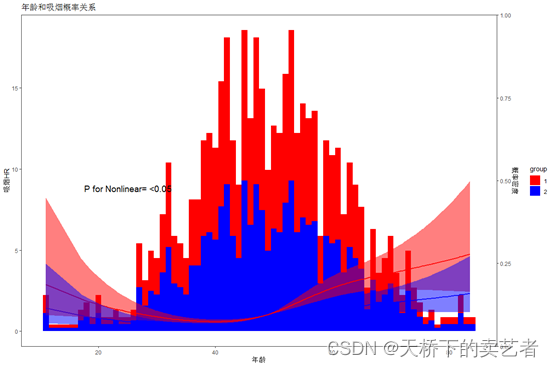``````ggrcs(data=dt,fit=fit,x="age",group="gender",
groupcol=c("red","blue"),histbinwidth=1,ribalpha=0.5,xlab ="年龄",
ylab="吸烟HR",title ="年龄和吸烟概率关系",leftaxislimit=c(0,1),liftname="概率密度",
P.Nonlinear=T,Pvalue="<0.05",xP.Nonlinear=25,yP.Nonlinear=18)
``````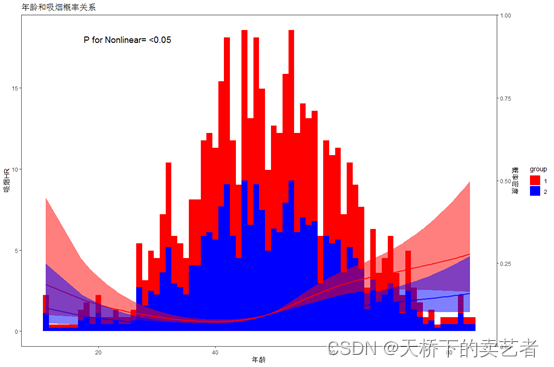``````library(foreign)
be <- read.spss("E:/r/test/Breast cancer survival agec.sav",
use.value.labels=F, to.data.frame=T)
be <- na.omit(be)
be\$ln_yesno<-as.factor(be\$ln_yesno)
``````

``````fit <-lrm(status ~ rcs(age, 4)+ln_yesno,data=be)
``````

``````ggrcs(data=be,fit=fit,x="age",group="ln_yesno",histbinwidth=1)
``````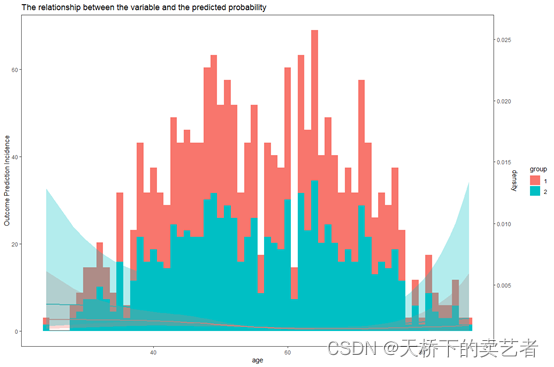``````ggrcs(data=be,fit=fit,x="age",group="ln_yesno",histbinwidth=1,groupcol=c("red","blue"),
xlab ="年龄",
ylab="吸烟HR",title ="年龄和吸烟概率关系",leftaxislimit=c(0,1),liftname="概率密度",
P.Nonlinear=T,Pvalue="<0.05",xP.Nonlinear=40,yP.Nonlinear=70)
``````